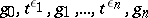# HNN-extension

In 1949, G. Higman, B.H. Neumann and H. Neumann [a4] proved several famous embedding theorems for groups using a construction later called the HNN-extension. The theory of HNN-groups is central to geometric and combinatorial group theory and should be viewed in parallel with amalgamated products (cf. also Amalgam of groups).

The easiest way to define an HNN-group is in terms of presentations of groups.

## Presentation of groups.

A presentation of a group $G$ is a pair $\langle X | R \rangle$ where $R$ is a subset of $F(X)$, the free group on the set $X$, and $G$ is isomorphic (cf. also Isomorphism) to the quotient group $F(X)/N(R)$, where $N(R)$ is the intersection of all normal subgroups of $F(X)$ containing $R$. The subgroup $N(R)$ is called the normal closure of $R$ in $F(X)$.

Given an arbitrary group $G$, there is an obvious homomorphism $\tau_G : F(G) \rightarrow G$ such that $\tau_G(g) = g$ for all $g \in G$. Clearly, $\langle G | \ker \tau_G \rangle$ is a presentation for $G$.

## HNN-extensions.

Suppose $\mu : A \rightarrow B$ is an isomorphism of subgroups of a group $G$ and $t$ is not in $G$. The HNN-extension of $G$ with respect to $\mu$ has presentation

\begin{equation*} \left\langle G \bigcup \{ t \} : ( \operatorname { ker } ( \tau _ { G } ) ) \bigcup \left\{ t ^ { - 1 } a ^ { - 1 } t \mu ( a ) : \forall a \in A \right\} \right\rangle. \end{equation*}

The generator $t$ is called the stable letter, $G$ the base group and $A$ and $B$ the associated subgroups of this HNN-extension. When $A = G$, the HNN-extension is called ascending.

Shorthand notation for the above group is $\langle G , t : t ^ { - 1 } A t = B , \mu \rangle$ or $G ^ { * } \mu$.

In [a4] it was shown that the mapping $G \rightarrow G ^ { * } \mu$ taking $g \rightarrow g$ for all $g \in G$ is a monomorphism. The rest of the normal form theorem for HNN-extensions was proved by J.L. Britton in 1963 [a1] (Britton's lemma): Let $g _ { 0 } , \ldots , g _ { n }$ be a sequence of elements of $G$ and let the letter, with or without subscripts, denote $\pm 1$. A sequencewill be called reduced if there is no consecutive subsequence $t ^ { - 1 } , g _ { i } , t$ with $g _ { i } \in A$ or $t , g _ { i } , t ^ { - 1 }$ with $g_i \in B$. For a reduced sequence and $n \geq 1$, the elementof $G _ { \mu } ^ { * }$ is different from the unit element.

In the original reference [a4], the following theorem is proved: Every group $G$ can be embedded in a group $G ^ { * }$ in which all elements of the same order are conjugate (cf. also Conjugate elements). In particular, every torsion-free group can be embedded in a group $G ^ { * * }$ with only two conjugacy classes. If $G$ is countable, so is $G ^ { * * }$. Also, every countable group $C$ can be embedded in a group $G$ generated by two elements of infinite order. The group $G$ has an element of finite order $n$ if and only if $C$ does. If $C$ is finitely presentable, then so is $G$.

For an excellent account of the history of HNN-extensions, see [a2]. See [a5], Chap. IV, for basic results and landmark uses of HNN-extensions, such as: the torsion theorem for HNN-extensions; the Collins conjugacy theorem for HNN-extensions; the construction of finitely-presented non-Hopfian groups (in particular, the Baumslag–Solitar group $\langle b , t : t ^ { - 1 } b ^ { 2 } t = b ^ { 3 } \rangle$ is non-Hopfian; cf. also Non-Hopf group); decompositions of $1$-relator groups; Stallings' classification of finitely-generated groups with more than one end in terms of amalgamated products and HNN-extensions; and Stallings' characterization of bipolar structures on groups.

HNN-extensions are of central importance in, e.g., the modern version of the Van Kampen theorem (based on topological results in [a8], [a9]); the Bass–Serre theory of groups acting on trees and the theory of graphs of groups (see [a7]); Dunwoody's accessibility theorem [a3]; and JSJ decompositions of groups [a6].

How to Cite This Entry:
HNN-extension. Encyclopedia of Mathematics. URL: http://encyclopediaofmath.org/index.php?title=HNN-extension&oldid=50616
This article was adapted from an original article by Mike Mihalik (originator), which appeared in Encyclopedia of Mathematics - ISBN 1402006098. See original article